# Intermediate Geometry : Midpoint Formula

## Example Questions

### Example Question #11 : Coordinate Geometry

A line segment has endpoints (8, 1) and (2, 5). What is the midpoint of this segment?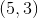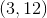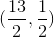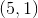Explanation:

Recall the formula for finding a midpoint of a line segment: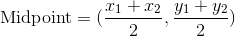The coordinates of the midpoint is just the average of the x-coordinates and the average of the y-coordinates.

Plug in the given points to find the midpoint of the line segment.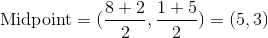### Example Question #11 : Lines

A line segment has endpoints at (10, 2) and (-10, -6). What is the midpoint of this line?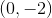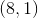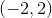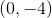Explanation:

Recall the formula for finding a midpoint of a line segment:The coordinates of the midpoint is just the average of the x-coordinates and the average of the y-coordinates.

Plug in the given points to find the midpoint of the line segment.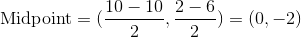### Example Question #13 : Coordinate Geometry

A line segments has endpoints at (-5, 1) and (-2, 9). What is the midpoint of this line segment?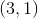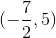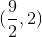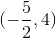Explanation:

Recall the formula for finding a midpoint of a line segment:The coordinates of the midpoint is just the average of the x-coordinates and the average of the y-coordinates.

Plug in the given points to find the midpoint of the line segment.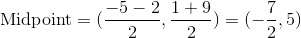### Example Question #14 : Coordinate Geometry

Find the midpoint of a line that has endpoints at (9, 2) and (11, 6).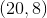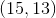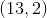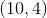Explanation:

Recall the formula for finding a midpoint of a line segment:The coordinates of the midpoint is just the average of the x-coordinates and the average of the y-coordinates.

Plug in the given points to find the midpoint of the line segment.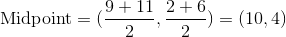### Example Question #15 : Coordinate Geometry

Find the midpoint of a line that has endpoints at (-15, 2) and (-2, 2).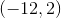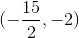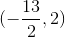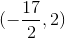Explanation:

Recall the formula for finding a midpoint of a line segment:The coordinates of the midpoint is just the average of the x-coordinates and the average of the y-coordinates.

Plug in the given points to find the midpoint of the line segment.### Example Question #16 : Coordinate Geometry

Find the midpoint of a line segment that has endpoints at (-9,-1) and (1,2).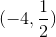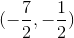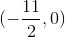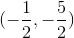Explanation:

Recall the formula for finding a midpoint of a line segment:The coordinates of the midpoint is just the average of the x-coordinates and the average of the y-coordinates.

Plug in the given points to find the midpoint of the line segment.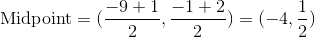### Example Question #17 : Coordinate Geometry

Find the midpoint of a line segment with endpoints at (10, 2) and (-2, -8).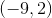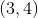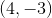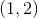Explanation:

Recall the formula for finding a midpoint of a line segment:The coordinates of the midpoint is just the average of the x-coordinates and the average of the y-coordinates.

Plug in the given points to find the midpoint of the line segment.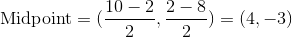### Example Question #18 : Coordinate Geometry

Find the midpoint of a line segment that has endpoints at (5,1) and (-3, -10).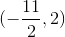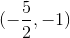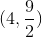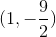Explanation:

Recall the formula for finding a midpoint of a line segment:The coordinates of the midpoint is just the average of the x-coordinates and the average of the y-coordinates.

Plug in the given points to find the midpoint of the line segment.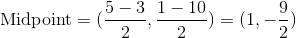### Example Question #19 : Coordinate Geometry

Find the midpoint of a line segment that has endpoints at (0.6, 9) and (-9.2, 7).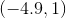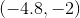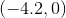Explanation:

Recall the formula for finding a midpoint of a line segment:The coordinates of the midpoint is just the average of the x-coordinates and the average of the y-coordinates.

Plug in the given points to find the midpoint of the line segment.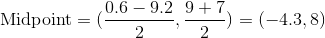### Example Question #20 : Coordinate Geometry

Find the midpoint of a line segment that has endpoints at (12, 12) and (-10, 24).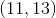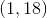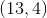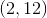Explanation:

Recall the formula for finding a midpoint of a line segment:The coordinates of the midpoint is just the average of the x-coordinates and the average of the y-coordinates.

Plug in the given points to find the midpoint of the line segment.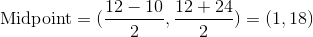### All Intermediate Geometry Resources